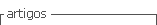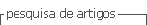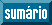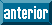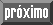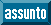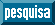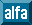## Artigo

•Citado por SciELO
•Acessos

•Citado por Google
•Similares em SciELO
•Similares em Google

## versão impressa ISSN 0120-419X

### Integración - UIS vol.36 no.2 Bucaramanga jul./dez. 2018

#### http://dx.doi.org/10.18273/revint.v36n2-2018006

Artículos originales

Multilinear analysis for discrete and periodic pseudo-differential operators in Lp-spaces

Análisis multilineal para operadores pseudodiferenciales periódicos y discretos en espacios Lp

Duván Cardonaa  *  , Vishvesh Kumarb

a Pontificia Universidad Javeriana, Department of Mathematics, Bogotá, Colombia.

b Indian Institute of Technology Delhi, Department of Mathematics, New Delhi-110016, India.

Abstract

In this note we announce our investigation on the L p properties for periodic and discrete multilinear pseudo-differential operators. First, we review the periodic analysis of multilinear pseudo-differential operators by showing classical multilinear Fourier multipliers theorems (proved by Coifman and Meyer, Tomita, Miyachi, Fujita, Grafakos, Tao, etc.) in the context of periodic and discrete multilinear pseudo-differential operators. For this, we use the periodic analysis of pseudo-differential operators developed by Ruzhansky and Turunen. The s-nuclearity, 0 < s ≤ 1, for the discrete and periodic multilinear pseudo-differential operators will be investigated. To do so, we classify those s-nuclear, 0 < s ≤ 1, multilinear integral operators on arbitrary Lebesgue spaces defined on σ-finite measures spaces. Finally, we present some applications of our analysis to deduce the periodic Kato-Ponce inequality and to examine the s-nuclearity of multilinear Bessel potentials as well as the s-nuclearity of periodic Fourier integral operators admitting suitable types of singularities.

MSC2010: 58J40, 47B10, 47G30, 35S30.

Key words: Pseudo-differential operator; discrete operator; periodic operator; nuclearity; boundedness; Fourier integral operator; multilinear analysis

Resumen

1. Introduction

The goal of this note is to announce the main results about the Lp-multilinear analysis developed by the authors in  for periodic and discrete pseudo-differential operators. These operators can be defined by using the multilinear Fourier transform as follows. If is a suitable function, then the periodic multilinear-pseudo-differential operator associated to m is the operator defined as

is the periodic multilinear Fourier transform of f. We have denoted by the space of smooth functions on the torus endowed with its usual Fréchet structure. On the other hand, if α: is a measurable function, then the discrete multilinear-pseudo-differential operator associated to α is the multilinear operator defined by

the discrete Fourier transform of gi. For r ≥ 2, these operators have been studied by V. Catană in . If r = 1, these quantization formulae can be reduced to the known expressions

and

Periodic pseudo-differential operators (see (3)) were defined by Volevich and Agranovich . The subsequent works of McLean , Turunen and Vainikko , and Ruzhansky and Turunen  developed a periodic analysis from Hörmander classes to applications to periodic equations, although the symbolic calculus was consistently developed by Ruzhansky and Turunen . Nevertheless, the references Ruzhansky and Turunen , , Cardona , , , Delgado  and Molahajloo and Wong , ,  provide some complementary results for the subject. Mapping properties for more general operators as periodic Fourier integral operators appear in Ruzhansky and Turunen  and Cardona, Messiouene and Senoussaoui .

In a more recent approach, pseudo-differential operators on (discrete pseudo-differential operators) were introduced by Molahajloo in , and some of its properties were developed in the last years in the references , , , , , , , . However, only the fundamental work L. Botchway, G. Kibiti, and M. Ruzhansky  includes properties about a discrete pseudo-differential calculus and applications to difference equations. The reference  discusses those relations of the theory of discrete pseudo-differential operators with important problems in number theory as the Waring problem and the hypothesis K * by Hooley.

An overview to the mapping properties for pseudo-differential operators on provides the expected results in the discrete and periodic setting. On these operators have the form

with the euclidean Fourier transform of f (see Hörmander ). The nuclearity of pseudo-differential operators on has been treated in Aoki and Rempala , . In a context closely related to our work, multilinear pseudo-differential operators have been treated in Bényi, Maldonado, Naibo, and Torres, , , Michalowski, Rule and Staubach, Miyachi and Tomita , , ,  and references therein. The multilinear analysis for multilinear Fourier multipliers

born with the works of Coifman and Meyer , , where the condition

for sufficiently many multi-indices α = (α12, · · · , ar), was proved to be sufficient for the boundedness of Ta from into provided that 1/p = 1/p1 + 1/p2 + · · · + 1/pr, and 1 ≤ pi, p < ∞. A generalization for this result was obtained by Tomita in , where it was proved that the multilinear Hörmander condition

implies the boundedness of Ta from into provided that 1/p = 1/p1 + 1/p2 + · · · + 1/pr, and 1 ≤ pi, p < ∞. The case r = 1 is known as the Hörmander-Mihlin theorem. These multilinear theorems have been generalized to Hardy spaces for suitable values of 0 < pi, p < ∞, in the works of Grafakos, Torres, Miyachi, Fujita, Tomita, Kenig, Stein, Muscalo, Thiele and Tao , , , , , , . The main novelty of this work is that we provide discrete and periodic analogues for these works in the multilinear setting.

This note is organized as follows. In section 2 we provide those results on the boundedness of pseudo-differential operators on and the torus. Later, in Section 3 we classify those s-nuclear multilinear integral operators on arbitrary σ-finite measure spaces and we apply this classification to periodic and discrete multilinear pseudo-differential operators.

2. Boundedness of pseudo-differential operators on 𝕋n and n

In this section we explain in detail the main results of our investigation on the bounded-ness of the multilinear operators considered. Our starting point is the following multilinear version of the Stein-Weiss multiplier theorem (see Theorem 3.8 of Stein and Weiss ). Sometimes we denote (x,ξ) := (x, ξ1, · · · , ξr) = x · (ξ1 + · · · + ξr).

Theorem 2.1. Let 1 <p < ∞ and let α: be a continuous bounded function. If the multilinear Fourier multiplier operator

extends to a bounded multilinear operator from into then the periodic multilinear Fourier multiplier

also extends to a bounded multilinear operator from into provided that

Moreover, there exists a positive constant C p such that the following inequality holds:

Remark 2.2. Theorem 2.1 can be proved in the following way. By the density of the trigonometric polynomials, we can prove that under the conditions of this theorem, we have the estimate

where the constant C does not dependent of every trigonometric polynomial Pi. For this, we will prove that

for some positive constant cn,r,p > 0. We will assume that

Observe that by linearity, we only need to prove (10) when for k and mi in The main step in our proof (see Cardona and Kumar ) is to show (10) and how it implies (9).

With the help of the previous result we prove the following fact. We use the notation

for all Now, we provide the following discrete version of the known result of Coifman and Meyer mentioned in the introduction.

Theorem 2.3. Let Tm be a periodic multilinear Fourier multiplier. Under the condition

the operator T m extends to a bounded multilinear operator from provided that

If we consider Fourier integral operators (FIOs) with periodic phases, we can recover the following multilinear version for FIOs of the multiplier theorem of Stein and Weiss.

Theorem 2.4. Let 1 < p < ∞ and let ф be a real valued continuous function defined on is a continuous bounded function, and the multilinear Fourier integral operator

extends to a bounded multilinear operator from into then the periodic multilinear Fourier integral operator

also extends to a bounded multilinear operator from into provided that

Moreover, there exists a positive constant CP such that

Now, we present some results about the boundedness of periodic multilinear pseudodifferential operators where explicit conditions on the multilinear symbols are considered.

Theorem 2.5. Let us assume that m satisfies the Hörmander condition of order s > 0,

Then the multilinear periodic pseudo-differential operator Tm associated with m extends to a bounded operator from provided that and

Remark 2.6. The proof of Theorem 2.5 is based on a suitable Littlewood-Paley decomposition of the symbol m. Indeed, we decompose m as

We prove that by assuming (11), we can decompose the operator Tm as

where every operator T mj is associated to the symbol m j, and we prove that the operator norm of every Tmj is less than multiplied by a factor proportional to We conclude our proof in  by observing that

The following theorem is an extension of the Coifman-Meyer result presented above in the multilinear pseudo-differential framework.

Theorem 2.7. Let us assume that m satisfies the discrete symbol inequalities

sup |A|1 1 A|2 ··· A|; m(xfe, ··· ,ξr )| < Cafe-H (14)

for all Then the periodic multilinear pseudo-differential operator Tm extends to a bounded operator from provided that

Remark 2.8. We prove Theorem 2.7 by observing that (14) implies (11). We develop this delicate argument in  where we use, among other things, the periodic analysis developed by Ruzhansky and Turunen.

The condition on the number of discrete derivatives in the preceding result can be relaxed if we assume regularity in x. We show it in the following theorem.

Theorem 2.9. Let Tm be aperiodic multilinear pseudo-differential operator. If m satisfies toroidal conditions of the type

whereextends to a bounded multilinear operator from provided that

Example 2.10. Theorem 2.7 applied to the bilinear operator

where Js is the periodic fractional derivative operator or the periodic Bessel potential of order implies the (well known) periodic Kato-Ponce inequality:

whereis the Laplacian on the torus.

Boundedness of discrete multilinear pseudo-differential operators. Our main results about the boundedness of discrete multilinear pseudo-differential operators are stated as follows.

Theorem 2.11. Let If σ satisfies the discrete inequality

for all β with then Tσ extends to a bounded operator from provided that 1 ≤ pj ≤ p ≤ ∞, and

The following result can be derived of the previous result with r = 1 and s = p.

Corollary 2.12. Let If σ satisfies the discrete inequality

3. s-Nuclearity, 0 < s ≤ 1, of pseudo-differential operators on 𝕋n and n

In this section we study the s-nuclearity, 0 < s ≤ 1 of multilinear discrete and periodic pseudo-differential operators. We prove Theorem 3.1 regarding the characterization of s-nuclear multilinear operators on abstract σ-finite measure spaces, and Theorem 3.2 and Theorem 3.3 regarding the characterization of s-nuclearity of periodic and discrete pseudo-differential operators. Although these theorems are multilinear extensions of the results due to Delgado , Delgado and Wong , JamalpourBirgani  and Ghaemi, JamalpourBirgani and Wong , we can recover their results from our results by considering r = 1. In order to study these multilinear operators admitting s-nuclear extensions, we prove the following multilinear version of a result by Delgado, on the nuclearity of integral operators on Lebesgue spaces (see , ). So, in the following multilinear theorem we characterize those s-nuclear (multilinear) integral operators on arbitrary (σ-finite) measure spaces (X,μ).

Theorem 3.1. Letίί), 1 ≤ i ≤ r and (Y, ν) be σ-finite measure spaces. Let 1 ≤ P i ,ρ < ∞, 1 ≤ i ≤ r and letbe such that for 1 ≤ i ≤ r. Let be a multilinear operator. Then T is a s-nuclear, 0 < s ≤ 1, operator if, and only if, there exist sequences {gn}n with gn = (gn1,gn2,...,gnr) and and LP(v), respectively, such that and for all f = (f1, f2,...,fr) ∈ LP1 (μ) χ LP2 (μ2) χ ··· χ LPr (μr ) we have

for almost every y ∈ Y.

Remark 3.2. The proof of Theorem 3.1 is based on an important lemma proved in [10, Lemma 4.1]. The proof of the if part of Theorem 3.1 follows using the definition of nuclear operators, Lemma 4.1 (iv) of  and the fact that Lp-convergence of a sequence implies the convergence of a sequence almost everywhere.

The only if part of Theorem 3.1 is a straightforward using the part (iv) of [10, Lemma 4.1] and applications of monotone convergence theorem of B. Levi and Lebesgue dominated convergence theorem.

This criterion applied to discrete and periodic operators gives the following characterizations (for the proof we refer the reader to ).

Theorem 3.3. Let a be a measurable function defined on . The multilinear pseudo-differential operator for all 1 ≤ i ≤ r, is a s -nuclear, 0 < s ≤ 1, operator if, and only if, the following decomposition holds:

Similarly, we can classify the s-nuclearity of periodic multilinear operators.

Theorem 3.4. Let m be a measurable function on Then the mutlilinear pseudo-differential operator is a s -nuclear, 0 < s ≤ 1, operator if, and only if, there exist two sequences {gk}k with for 1 ≤ ir and respectively, such that and

Now, we present the following sharp result on the s-nuclearity of periodic Fourier integral operators.

Theorem 3.5. Let us consider the real-valued function Let us consider the Fourier integral operator

with symbol satisfying the summability condition

Then A extends to a s-nuclear, 0 < s ≤ 1, operator from into provided that 1 ≤ p j < ∞, and 1 ≤ p ≤ ∞.

Remark 3.6. The proof of Theorem 3.5 follows using Theorem 3.1 by considering the function

the functional

and their estimates

Example 3.7. In order to illustrate the previous conditions, we consider the multilinear Bessel potential. This can be introduced as follows. Consider the periodic multilinear Laplacian denoted by

For r = 1, we recover the usual periodic Laplacian

The multilinear Bessel potential of order

can be defined by the Fourier analysis associated to the torus as

From the estimate

Theorem 3.5 applied to implies that the multilinear Bessel potential extends to a s-nuclear operator from into for all 1 ≤ pj < ∞ and 1 ≤ p ≤ ∞, provided that

This conclusion is sharp, in the sense that if we restrict our analysis to r = 1 and p1 = ρ = 2, the operatorextends to a s-nuclear operator on if, and only if,

Example 3.8. Now, we consider FIOs with symbols admitting some type ofsingularity at the origin. In this general context, let us choose a sequence Let us consider the symbol

we consider the Fourier integral operator associate to α(·, ·),

the condition

0 < ρ < n/p

implies that the periodic Fourier integral operator A extends to a s -nuclear multilinear operator from for all 1 ≤ pj < ∞ and 1 ≤ p ≤ ∞. In fact, by Theorem 3.5, we only need to verify that

But, for every ρ > 0, this happens if, and only if, 0 < ρ < n/p.

Acknowledgment

Vishvesh Kumar thanks the Council of Scientific and Industrial Research, India, for its senior research fellowship. Duván Cardona was partially supported by Pontificia Universidad Javeriana, Mathematics Department. We would like to thank the anonymous referee for his/her careful evaluation which helped to improve the presentation of this work.

References

 Agranovich M.S., "Spectral properties of elliptic pseudo-differential operators on a closed curve", Funct. Anal. Appl. 13 (1971), 279-281. [ Links ]

 Aoki S., "On the boundedness and the nuclearity of pseudo-differential operators", Comm. Partial Diff. Equations 6 (1981), No. 8, 849-881. [ Links ]

 Bényi A., Bernicot F., Maldonado D., Naibo V. and Torres R., "On the Hörmander classes of bilinear pseudo-differential operators II", In diana Univ. Math. J. 62 (2013), 1733-1764. [ Links ]

 Bényi A., Maldonado D., Naibo V. and Torres R., "On the Hörmander classes of bilinear pseudodifferential operators", Integral Equ. Oper. Theory 67 (2010), 341-364. [ Links ]

 Botchway L., Kibiti G. and Ruzhansky M., "Difference equations and pseudo-differential operators on Zn", arXiv:1705.07564. [ Links ]

 Cardona D. "Estimativos L2 para una clase de operadores pseudodiferenciales definidos en el toro", Rev. Integr. Temas Mat. 31 (2013), No. 2, 142-157. [ Links ]

 Cardona D., "Weak type (1, 1) bounds for a class of periodic pseudo-differential operators", J. Pseudo-Differ. Oper. Appl. 5 (2014), No. 4, 507-515. [ Links ]

 Cardona D., "On the boundedness of periodic pseudo-differential operators", Monatsh. Math. 185 (2017), No. 2, 189-206. [ Links ]

 Cardona D., "Pseudo-differential operators on Zn with applications to discrete fractional integral operators", arXiv:1803.00231. [ Links ]

 Cardona D. and Kumar V., "Lp-boundedness and Lp-nuclearity of multilinear pseudo-differential operators on Zn and the torus Tn", arXiv:1809.08380. [ Links ]

 Cardona D., Messiouene R. and Senoussaoui A., "Lp-bounds for periodic Fourier integral operators (Fourier series operators)", arXiv:1807.09892. [ Links ]

 Catana V., "Lp-boundedness of Multilinear Pseudo-differential Operators on Zn and Tn", Math. Model. Nat. Phenom. 9 (2014), No. 5, 17-38. [ Links ]

 Coifman R. and Meyer Y., "On commutators of singular integrals and bilinear singular integrals", Trans. Amer. Math. Soc. 212 (1975), 315-331. [ Links ]

 Coifman R. and Meyer Y., Ondelettes et operateurs III. Operateurs multilineaires, Hermann, Paris, (1991). [ Links ]

 Delgado J., "Lp bounds for pseudo-differential operators on the torus", Oper. Theory Adv. Appl. 231 (2012), 103-116. [ Links ]

 Delgado J., "A trace formula for nuclear operators on Lp", Pseudo-Differential Operators: Complex Analysis and Partial Differential Equations, Operator Theory: Advances and Applications, in: Schulze, B.W., Wong, M.W. (eds.) 205, Birkhäuser, Basel (2010), 181-193. [ Links ]

 Delgado J. and Wong M.W., "Lp-nuclear pseudo-differential operators on Z and S1", Proc. Amer. Math. Soc. 141 (2013), No. 11, 3935-3944. [ Links ]

 Delgado J., "The trace of nuclear operators on Lp(µ) for σ-finite Borel measures on second countable spaces", Integr. Equ. Oper. Theory 68 (2010), No. 1, 61-74. [ Links ]

 Fujita M. and Tomita N., "Weighted norm inequalities for multilinear Fourier multipliers",Trans. Amer. Math. Soc . 364 (2012), No. 12, 6335-6353. [ Links ]

 Ghaemi M. B., Jamalpour Birgani M. and Wong M.W., "Characterizations of nuclear pseudo-differential operators on S1 with applications to adjoints and products",J. Pseudo-Differ. Oper. Appl . 8 (2017), No. 2, 191-201. [ Links ]

 Grafakos L., Miyachi A. and Tomita N., "On multilinear Fourier multipliers of limited smoothness", Canad. J. Math. 65 (2013), 299-330. [ Links ]

 Grafakos L. and Si Z., "The Hörmander multiplier theorem for multilinear operators", J. Reine Angew. Math. 668 (2012), 133-147. [ Links ]

 Grafakos L. and Torres R., "Discrete decompositions for bilinear operators and almost diagonal conditions",Trans. Amer. Math. Soc . 354 (2012), 1153-1176. [ Links ]

 Grafakos L. and Torres R., "Multilinear Calderón-Zygmund theory", Adv. Math. 165 (2002), 124-164. [ Links ]

 Hörmander L., The Analysis of the linear partial differential operators Vol. III, IV SpringerVerlag, (1985). [ Links ]

 Jamalpour Birgani M., "Characterizations of Nuclear Pseudo-differential Operators on Z with some Applications",Math. Model. Nat. Phenom . 13 (2018), 13-30. [ Links ]

 Kenig C. and Stein E., "Multilinear estimates and fractional integration", Math. Res. Lett. 6 (1999), 1-15. [ Links ]

 Kumar V., "Pseudo-differential operators on homegeneous spaces of Compact and Hausdorff groups", to appear in Forum Mathematicum, (2018). [ Links ]

 Mclean W.M., "Local and Global description of periodic pseudo-differential operators", Math. Nachr. 150 (191), 151-161. [ Links ]

 Michalowski N., Rule D. and Staubach W., "Multilinear pseudodifferential operators beyond Calderón-Zygmund operators", J. Math. Anal. Appl. 414 (2014), 149-165. [ Links ]

 Miyachi A. and Tomita N., "Minimal smoothness conditions for bilinear Fourier multipliers", Rev. Mat. Iberoam. 29 (2013), 495-530. [ Links ]

 Miyachi A. and Tomita N. "Calderón-Vaillancourt type theorem for bilinear operators", Indiana Univ. Math. J . 62 (2013), 1165-1201. [ Links ]

 Miyachi A. and Tomita N. "Bilinear pseudo-differential operators with exotic symbols", to appear in Ann. Inst. Fourier (Grenoble), arXiv:180106744. [ Links ]

 Molahajloo S., "A characterization of compact pseudo-differential operators on S1",Oper. Theory Adv. Appl . 213 (2011), 25-29. [ Links ]

 Molahajloo S. and Wong M.W., "Pseudo-differential Operators on S1", New developments in pseudo-differential operators, Eds. L. Rodino and M.W. Wong. 297-306 (2008). [ Links ]

 Molahajloo S. and Wong M.W., "Ellipticity, Fredholmness and spectral invariance of pseudo-differential operators on S1" J. Pseudo-Differ. Oper. Appl . 1 (2010), No. 2, 183-205. [ Links ]

 Muscalu C., Tao T. and Thiele C., "Multilinear operators given by singular multipliers", J. Amer. Math. Soc. 15 (2002), 469-496. [ Links ]

 Rabinovich V.S., "Exponential estimates of solutions of pseudo-differential equations on the lattice (µZ)n, applications to the lattice Schrödinger and Dirac operators",J. Pseudo-Differ. Oper. Appl . 1 (2010), No. 2, 233-253. [ Links ]

 Rabinovich V.S., "Wiener algebra of operators on the lattice (µZ)n depending on the small parameter μ > 0", Complex Var. Elliptic Equ. 58 (2013), No. 6, 751-766. [ Links ]

 Rabinovich V.S. and Roch S., "The essential spectrum of Schrödinger operators on lattices", J. Phys. A. 39 (2006), No. 26, 8377-8394. [ Links ]

 Rabinovich V.S. and Roch S., "Essential spectra and exponential estimates of eigenfunctions of lattice operators of quantum mechanics", J. Phys. A. 42 (2009), No. 38, 385207. [ Links ]

 Rempala J.A., "On a proof of the boundedness and nuclearity of pseudodifferential operators in Rn", Annales Polonici Mathematici 52 (1990), 59-65. [ Links ]

 Rodriguez C.A., "Lp-estimates for pseudo-differential operators on Zn",J. Pseudo-Differ. Oper. Appl . 2 (2011), 367-365. [ Links ]

 Ruzhansky M. and Turunen V., "Quantization of Pseudo-Differential Operators on the Torus", J. Fourier Anal. Appl. 16 (2010), 943-982. [ Links ]

 Ruzhansky M. and Turunen V., Pseudo-differential Operators and Symmetries: Background Analysis and Advanced Topics, Birkhaüser-Verlag, Basel, (2010). [ Links ]

 Tomita N., "A Hörmander type multiplier theorem for multilinear operators", J. Funct. Anal. 259 (2010), 2028-2044. [ Links ]

 Turunen V. and Vainikko G., "On symbol analysis of periodic pseudodifferential operators, Z. Anal. Anwendungen 17 (1998), 9-22. [ Links ]

 Stein E. and Weiss G., Introduction to Fourier analysis on Euclidean spaces, Princeton University Press, Princeton, N.J. (1971). [ Links ]

To cite this article:] D. Cardona, V. Kumar, Multilinear analysis for discrete and periodic pseudo-differential operators in Lp-spaces, Rev. Integr. temas mat. 36 (2018), No. 2, 151-164. doi: 10.18273/revint.v36n2-2018006.

Received: September 24, 2018; Accepted: November 29, 2018

* E-mail: duvanc306@gmail.comThis is an open-access article distributed under the terms of the Creative Commons Attribution License